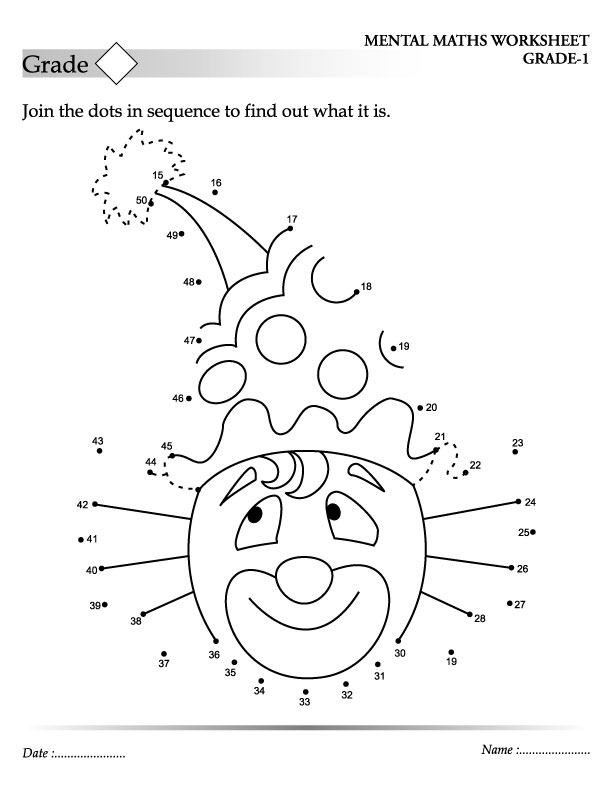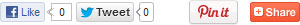# Home

### Join the dots in sequence to find out what it is

Page of 39Page of 39Draw some more objects like the given oneColour the box which has highest numberMatch the followingTime related questionsFun with moneyColour the balloons in red and bubbles in yellowColour the thinnest, biggest and farthest objectsColor the capMatch and check are there enough plates for each cupFun with numbersTens and onesAddition and subtractionHow many are remainingHow many leftAdd and write the answers in given spaceMatch with correct answersCount, add and writeTick the correct count in each boxAltogether�how manyAfter and before�Make the number chainEncircle the highest number in each rowWrite the number names of the followingWhat comes nextBackward countingWrite in increasing orderMatch the numerals with their number namesWrite the higher number in the star in each rowWrite the numbers which come in betweenWhat comes after-What comes beforeWhat comes after, What comes beforeDraw a line to match the number to the objectsCount and color the correct number boxMatch the objects with their correct numberAltogether--how manyCount and cross the wrong numbersCount and tick the correct numberCount and write the correct number in the boxCount and write